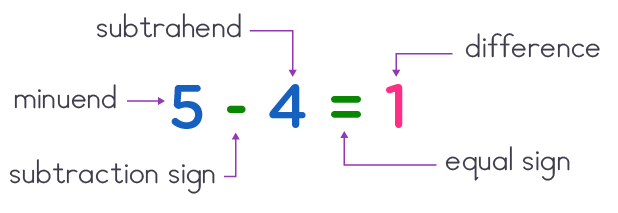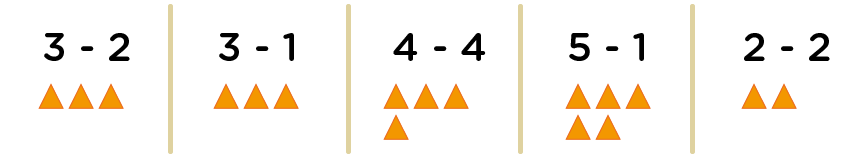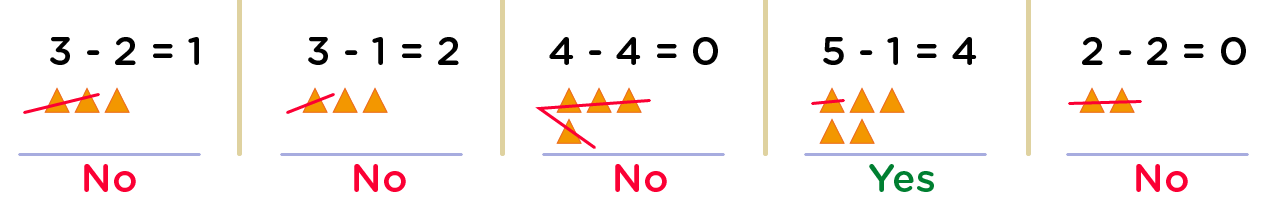# Subtraction up to 5 Worksheets for Kindergarten - Free Printable Subtraction Math for Kindergarten

## Subtraction for Kindergarten PDF

We use subtraction every day in our daily activities, thus an important math skills which kids must learn at an early age. For this reason, subtraction up to 5 worksheets for kindergarten is all about molding kinders with outstanding exercises for them to effectively know how to easily take one or more numbers away from another.

• Subtraction up to 5
Print it...
• Subtract numbers up to 5
Print it...

## Subtraction facts

For your kinders to become math wizards and proficient in subtraction in particular, simply grab our free printable subtraction math for kindergarten. This worksheet will instill excitement into kinders math lives as they will tackle subtraction problems only with basic numbers up to 5.

Before moving on to solving subtraction sentences in our subtraction for kindergarten pdf, it is very important for kids to have an in-depth understanding of parts of a subtraction sentence.

They include;

• The subtrahend, which is the number to be taken away, and
• The difference, which is the part left after subtracting. It comes after the equal sign (=)

An example of a subtraction sentence is →         2      -     1    =    1

Parts of a subtraction sentence includeEqually important is the subtraction sign, also referred to as “take away” or “minus”. It’s represented thus “

### Fun and easy to solve – subtraction sentences: basic numbers up to 5

Subtraction up to 5 worksheets for kindergarten has very fun and easy to solve subtraction exercises. These subtraction sentences: basic numbers up to 5 will enable kids to quickly grasp the rules of subtraction with less stress.

However, by using these our subtraction for kindergarten pdf worksheets, kids will be kept engaged as they find it exciting and anxious to know what is “left”.

Furthermore, in order to gain kid’s attention and concentration, we have well formulated exercises that will recreate kid’s logical thinking progression.

An example of one of such exercises is how do you make 4?   Circle the correct subtraction sentence. Hey kiddos, from a given multiple of subtraction sentences, let’s find out below the method to use to obtain the correct subtraction sentence.

### Example: how do you make 4? Circle the correct subtraction sentence.

3 - 2          3 - 1          4 - 4          5 - 1          2 - 2

Hey kids, as earlier said, we are going to find out which one of the above subtraction sentences can make 4. So, how do you make 4?

• Firstly, we will represent all the minuends in each subtraction sentence with objects.• Next, we’ll now take away by crossing out all the objects that represent the subtrahend in each multiplication sentence
• After crossing all the objects that represent the subtrahend, we can now count the number of uncrossed objects in each case. It is awesome to note that the number of objects uncrossed is the difference.WOW! This is so perfect

• Now, in respect to our question how do you make 4? We’ll look for the subtraction sentence whose difference is 4, then of course circle it.

Oooops! I got it. The correct subtraction sentence is → 5 - 1

BRAVOO! It’s so simple kiddos. Isn’t it? Have fun ok. And continue solving your subtraction problems diligently.

•